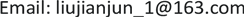1海军装备部装备采购中心，北京

2中国舰船研究设计中心，湖北 武汉

3大连船舶重工集团有限公司，辽宁 大连1. 引言

2. 热应力数学模型

1) 轴对称运动微分方程

{ ∂ σ x ∂ x + ∂ τ r x ∂ x + τ r x r + X = ρ ∂ 2 u ∂ t 2 ∂ σ r ∂ r + ∂ τ x r ∂ x + σ r − σ θ r + R = ρ ∂ 2 υ ∂ t 2 (1)

2) 几何方程

{ ε } = [ ε x , ε r , ε θ , ε x r ] T = [ ∂ u ∂ x , ∂ υ ∂ r , υ r , ∂ υ ∂ x + ∂ u ∂ r ] T (2)

3) 物理方程

{ ε } = [ ε x 0 , ε r 0 , ε θ 0 , ε x r 0 ] T = [ α Δ T , α Δ T , α Δ T , 0 ] T (3)

{ σ x = E ( 1 + μ ) ( 1 − 2 μ ) [ ( 1 + μ ) ( ε x − ε x 0 ) + μ ( ε r − ε r 0 ) + μ ( ε θ − ε θ 0 ) ] σ r = E ( 1 + μ ) ( 1 − 2 μ ) [ μ ( ε x − ε x 0 ) + ( 1 − μ ) ( ε r − ε r 0 ) + μ ( ε θ − ε θ 0 ) ] σ θ = E ( 1 + μ ) ( 1 − 2 μ ) [ μ ( ε x − ε x 0 ) + μ ( ε r − ε r 0 ) + ( 1 − μ ) ( ε θ − ε θ 0 ) ] τ x y = E 2 ( 1 + μ ) ν x y (4)

3. 几何模型

4. 热应力计算及分析

5. 疲劳分析

6. 结论

1) 阀门能够承受高温载荷，计算得到的主要承压件均低于材料的许用应力，满足强度要求。

2) 该阀门可在设计工况下启闭144,000次，保证了阀门承压边界的完整性。